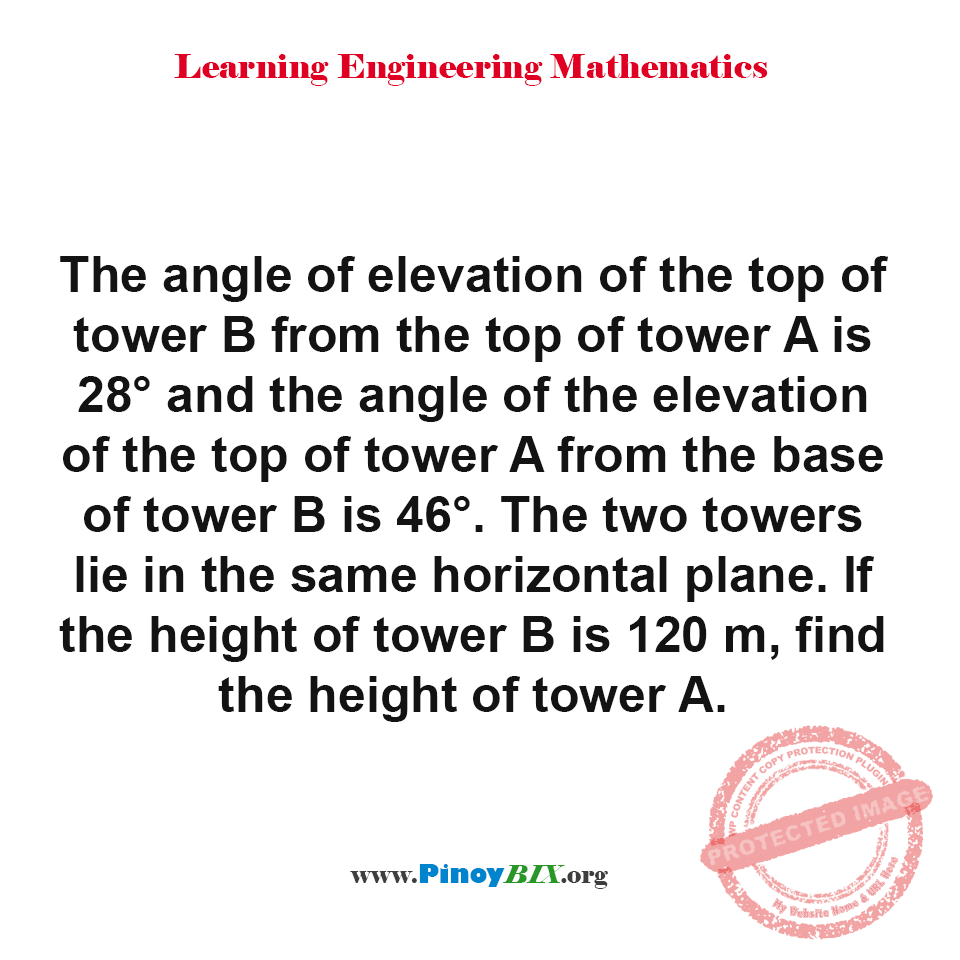# Solution: If the height of tower B is 120 m, find the height of tower A

(Last Updated On: March 2, 2019)#### Problem Statement: CE Board November 1997

The angle of elevation of the top of tower B from the top of tower A is 28° and the angle of the elevation of the top of tower A from the base of tower B is 46°. The two towers lie in the same horizontal plane. If the height of tower B is 120 m, find the height of tower A.

• A. 66.3 m
• B. 79.3 m
• C. 87.2 m
• D. 90.7 m

The height of tower A is 79.3 m.

Solution:

### Latest Problem Solving in Plane Trigonometry Problems

More Questions in: Plane Trigonometry Problems

#### Online Questions and Answers in Plane Trigonometry Problems

Solution: If the height of tower B is 120 m, find the height of tower A
5 (100%) 1 vote

#### One Response

1.messi